# 格林公式在微分方程中的应用Application of Green’s Formula in Differential Equations

DOI: 10.12677/AAM.2018.79137, PDF, HTML, XML, 下载: 836  浏览: 3,694

Abstract: This paper investigates the Green’s function solution of common non-homogeneous ordinary dif-ferential equations and partial differential equations. We first obtain Green’s functions according to the physical meanings of special partial differential equations. Then we yield their solutions. Considering non-homogeneous ordinary differential equations with insignificant physical meanings, this paper begins with the simplest first-order linear equations. Furthermore, the Green’s function of higher-order linear equations is derived.

1. 引言

1828年，乔治·格林(1793~1841)发表了《论数学分析在电磁理论上的应用》  ，在这篇著作中，格林试图确定由特定电势的导体在真空中的电势。用已知的符号，我们可以说格林想要得到在区域V中且满足特定边界条件S， ${\nabla }^{2}u=-f$ 的解。

${\nabla }^{2}G\left(r,{r}_{0}\right)=-4\text{π}\delta \left(r-{r}_{0}\right)$ (1)

${\iiint }_{V}\left(\phi {\nabla }^{2}\chi -\chi {\nabla }^{2}\phi \right)\text{d}V={∯}_{S}\left(\phi \nabla \chi -\chi \nabla \phi \right)\cdot n\text{d}S$ (2)

${\iiint }_{V}G{\nabla }^{2}u\text{d}V+{∯}_{S}G\nabla u\cdot n\text{d}S={\iiint }_{V}u{\nabla }^{2}G\text{d}V+{∯}_{S}u\nabla G\cdot n\text{d}S-4\text{π}u\left(r0\right)$

$u\left(r\right)=\frac{1}{\text{4π}}{∯}_{S}\stackrel{¯}{u}\nabla G\cdot n\text{d}S$

2. 格林公式

Gaussy公式：设空间闭域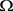是由光滑或分段光滑的双侧封闭曲面 $\Sigma$ 所围成，函数 $P\left(x,y,z\right)$$Q\left(x,y,z\right)$$R\left(x,y,z\right)$ 上具有一阶连续偏导数，则成立如下Gauss公式：

${\iiint }_{\Omega }\left(\frac{\partial P}{\partial x}\text{+}\frac{\partial Q}{\partial y}\text{+}\frac{\partial R}{\partial z}\right)\text{d}\Omega ={∯}_{\Sigma }\left[P\mathrm{cos}\left(n,x\right)+Q\mathrm{cos}\left(n,y\right)+R\mathrm{cos}\left(n,z\right)\right]\text{d}S$ (3)

2.1. 第一格林公式

$P=u\frac{\partial v}{\partial x},Q=u\frac{\partial v}{\partial y},R=u\frac{\partial v}{\partial z}$

$\begin{array}{l}{\iiint }_{\Omega }\left(\frac{\partial P}{\partial x}\text{+}\frac{\partial Q}{\partial y}\text{+}\frac{\partial R}{\partial z}\right)\text{d}\Omega \text{\hspace{0.17em}}={\iiint }_{\Omega }\left(\frac{\partial }{\partial x}\left(u\frac{\partial v}{\partial x}\right)\text{+}\frac{\partial }{\partial y}\left(u\frac{\partial v}{\partial y}\right)\text{+}\frac{\partial }{\partial z}\left(u\frac{\partial v}{\partial z}\right)\right)\text{d}x\text{d}y\text{d}z\\ \text{\hspace{0.17em}}\text{\hspace{0.17em}}\text{\hspace{0.17em}}\text{\hspace{0.17em}}\text{\hspace{0.17em}}\text{\hspace{0.17em}}\text{\hspace{0.17em}}\text{\hspace{0.17em}}\text{\hspace{0.17em}}\text{\hspace{0.17em}}\text{\hspace{0.17em}}\text{\hspace{0.17em}}\text{\hspace{0.17em}}\text{\hspace{0.17em}}\text{\hspace{0.17em}}\text{\hspace{0.17em}}\text{\hspace{0.17em}}\text{\hspace{0.17em}}\text{\hspace{0.17em}}\text{\hspace{0.17em}}\text{\hspace{0.17em}}\text{\hspace{0.17em}}\text{\hspace{0.17em}}\text{\hspace{0.17em}}\text{\hspace{0.17em}}\text{\hspace{0.17em}}\text{\hspace{0.17em}}\text{\hspace{0.17em}}\text{\hspace{0.17em}}\text{\hspace{0.17em}}\text{\hspace{0.17em}}\text{\hspace{0.17em}}={∯}_{\Sigma }\left[u\frac{\partial v}{\partial x}\mathrm{cos}\left(n,x\right)+u\frac{\partial v}{\partial y}\mathrm{cos}\left(n,y\right)+u\frac{\partial v}{\partial z}\mathrm{cos}\left(n,z\right)\right]\text{d}S\end{array}$

$\frac{\partial v}{\partial n}=\frac{\partial v}{\partial x}\mathrm{cos}\left(n,x\right)+\frac{\partial v}{\partial y}\mathrm{cos}\left(n,y\right)+\frac{\partial v}{\partial z}\mathrm{cos}\left(n,z\right)$${\iiint }_{\Omega }u\Delta v\text{d}x\text{d}y\text{d}z={∯}_{\Sigma }u\frac{\partial v}{\partial n}\text{d}S-{\iiint }_{\Omega }\nabla u\cdot \nabla v\text{d}x\text{d}y\text{d}z$ (4)

2.2. 第二格林公式

${\iiint }_{\Omega }v\Delta u\text{d}x\text{d}y\text{d}z={∯}_{\Sigma }v\frac{\partial u}{\partial n}\text{d}S-{\iiint }_{\Omega }\nabla v\cdot \nabla u\text{d}x\text{d}y\text{d}z$ (5)

 (6)

3. 格林函数

3.1. 基本积分公式

$u\left({M}_{0}\right)=\frac{1}{\text{4π}}{\iint }_{\Sigma }\left[\frac{1}{{r}_{M{M}_{0}}}\frac{\partial u}{\partial n}-u\frac{\partial }{\partial n}\left(\frac{1}{{r}_{M{M}_{0}}}\right)\right]\text{d}S-\frac{1}{\text{4π}}{\iiint }_{\Omega }\frac{\Delta u}{{r}_{M{M}_{0}}}\text{d}x\text{d}y\text{d}z$

$u\left({M}_{0}\right)=\frac{1}{4\text{π}}{\iint }_{\partial \Omega }\left[\frac{1}{{r}_{M{M}_{0}}}\frac{\partial u}{\partial n}-u\frac{\partial }{\partial n}\left(\frac{1}{{r}_{M{M}_{0}}}\right)\right]\text{d}S.$ (7)

3.2. 格林函数的定义

$\left\{\begin{array}{l}\Delta u=0,\left(x,y,z\right)\in \Omega \\ u|{}_{\partial \Omega }=f\left(x,y,z\right),\left(x,y,z\right)\in \partial \Omega \end{array}$ (8)

${\iint }_{\partial \Omega }\left(u\frac{\partial v}{\partial n}-v\frac{\partial u}{\partial n}\right)\text{d}S=0$ (9)

(7) + (9)，得

$u\left({M}_{0}\right)={\iint }_{\partial \Omega }\left[\left(\frac{1}{4\text{π}{r}_{M{M}_{0}}}-v\right)\frac{\partial u}{\partial n}-u\frac{\partial }{\partial n}\left(\frac{1}{4\text{π}{r}_{M{M}_{0}}}-v\right)\right]\text{d}S$

$v|{}_{\partial \Omega }=\frac{1}{4\text{π}{r}_{M{M}_{0}}},M\in \partial \Omega$

$u\left({M}_{0}\right)=-{\iint }_{\partial \Omega }u\frac{\partial }{\partial n}\left(\frac{1}{4\text{π}{r}_{M{M}_{0}}}-v\right)\text{d}S$

$G\left(M,{M}_{0}\right)=\frac{1}{4\text{π}{r}_{M{M}_{0}}}-v$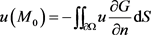$u\left({M}_{0}\right)=-{\iint }_{\partial \Omega }f\frac{\partial G}{\partial n}\text{d}S$ (10)

$G\left(M,{M}_{0}\right)$ 为Laplace方程Dirichlet问题在区域 $\Omega$ 上的格林函数。

4. 格林函数的性质

5. 格林函数在偏微分方程中的应用

$G\left(M,{M}_{0}\right)=\frac{1}{4\text{π}{r}_{M{M}_{0}}}-v\left(M,{M}_{0}\right)$

5.1. 半空间的Green函数及Dirichlet边值问题

$z>0$ 半空间的Green函数，并求解下列Dirichlet边值问题

$\left\{\begin{array}{l}\Delta u\left(x,y,z\right)=0,-\infty 0\\ u\left(x,y,0\right)=f\left(x,y\right),-\infty (11)

$G\left(M,{M}_{0}\right)=\frac{1}{4\text{π}}\left(\frac{1}{{r}_{M{M}_{0}}}-\frac{1}{{r}_{M{M}_{1}}}\right)$

$\begin{array}{c}{\frac{\partial G}{\partial n}|}_{z=0}={-\frac{\partial G}{\partial z}|}_{z=0}\\ ={\frac{1}{4\text{π}}\left[\frac{z-{z}_{0}}{{\left[{\left(x-{x}_{0}\right)}^{2}+{\left(y-{y}_{0}\right)}^{2}+{\left(z-{z}_{0}\right)}^{2}\right]}^{\frac{3}{2}}}-\frac{z+{z}_{0}}{{\left[{\left(x-{x}_{0}\right)}^{2}+{\left(y-{y}_{0}\right)}^{2}+{\left(z+{z}_{0}\right)}^{2}\right]}^{\frac{3}{2}}}\right]|}_{z=0}\\ =\frac{-1}{2\text{π}}\frac{{z}_{0}}{{\left[{\left(x-{x}_{0}\right)}^{2}+{\left(y-{y}_{0}\right)}^{2}+{z}_{0}^{2}\right]}^{\frac{3}{2}}}\end{array}$ (12)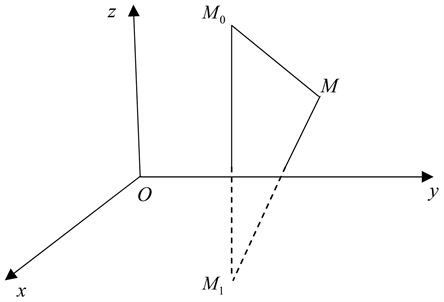Figure 1. The mirror image method

$u\left({M}_{0}\right)=\frac{{z}_{0}}{2\text{π}}{\int }_{-\infty }^{+\infty }{\int }_{-\infty }^{+\infty }\frac{f\left(x,y\right)}{{\left[{\left(x-{x}_{0}\right)}^{2}+{\left(y-{y}_{0}\right)}^{2}+{z}_{0}^{2}\right]}^{\frac{3}{2}}}\text{d}x\text{d}y$

5.2. 球体区域的Green函数及Dirichlet边值问题

$\left\{\begin{array}{l}\Delta u\left(x,y,z\right)=0,\left(x,y,z\right)\in \Omega \\ {u|}_{\Gamma }=f\left(x,y,z\right),\left(x,y,z\right)\in \Gamma \end{array}$ (13)

${r}_{0}{r}_{1}={R}^{2}$ (14)

$\frac{{r}_{M{}_{1}P}}{{r}_{{M}_{0}P}}=\frac{R}{{r}_{0}}$

$v\left(M,{M}_{0}\right)=\frac{R}{4\text{π}{r}_{0}{r}_{M{M}_{1}}}$

$G\left(M,{M}_{0}\right)=\frac{1}{4\text{π}{r}_{M{M}_{0}}}-\frac{1}{4\text{π}{r}_{0}{r}_{M{M}_{1}}}$ (15)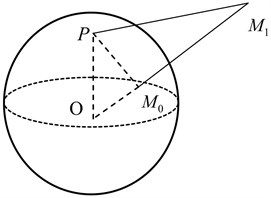Figure 2. Sphere area

${r}_{{M}_{0}M}^{2}={r}_{0}^{2}+{r}^{2}-2{r}_{0}r\mathrm{cos}a,\text{\hspace{0.17em}}{r}_{M{M}_{1}}^{2}={r}_{1}^{2}+{r}^{2}-2{r}_{1}r\mathrm{cos}a$

$G\left(M,{M}_{0}\right)=\frac{1}{\text{4π}}\left(\frac{1}{\sqrt{{r}_{0}^{2}+{r}^{2}-2{r}_{0}r\mathrm{cos}a}}-\frac{R}{\sqrt{{R}^{4}+{r}_{0}^{2}{r}^{2}-2{R}^{2}{r}_{0}r\mathrm{cos}a}}\right)$

$\begin{array}{c}{\frac{\partial G}{\partial n}|}_{\Gamma }={\frac{\partial G}{\partial n}|}_{r=R}={-\frac{1}{\text{4π}}\left(\frac{r-{r}_{0}\mathrm{cos}a}{{\left({r}_{0}^{2}+{r}^{2}-2{r}_{0}r\mathrm{cos}a\right)}^{3/2}}-\frac{R\left({r}_{0}^{2}r-{R}^{2}{r}_{0}\mathrm{cos}a\right)}{{\left({R}^{4}+{r}_{0}^{2}{r}^{2}-2{R}^{2}{r}_{0}r\mathrm{cos}a\right)}^{3/2}}\right)|}_{r=R}\\ =-\frac{1}{4\text{π}R}\frac{{R}^{2}-{r}_{0}^{2}}{{\left({R}^{2}+{r}_{0}^{2}-2Rr\mathrm{cos}a\right)}^{3/2}}\end{array}$

$u\left({M}_{0}\right)=\frac{1}{4\text{π}R}{\iint }_{\Gamma }f\left(x,y,z\right)\frac{{R}^{2}-{r}_{0}^{2}}{{\left({R}^{2}+{r}_{0}^{2}-2R{r}_{0}\mathrm{cos}a\right)}^{3/2}}\text{d}S$

6. 格林函数在常微分方程中的应用

6.1. 预备知识

$\delta$ 函数是由物理学家狄拉克首先引进的。在数学上，可以把 $\delta$ 函数看成广义函数。传统意义上， $\delta$ 函数的定义  是

$\delta \left(x\right)=\left\{\begin{array}{l}0,x\ne 0\\ \infty ,x=0\end{array}$

${\int }_{-\infty }^{+\infty }\delta \left(x\right)\text{d}x=1$ .

$\delta$ 函数一般具有下列性质  ：

1) ${\int }_{{\text{x}}_{1}}^{{x}_{2}}f\left(x\right)\delta \left(x\right)\text{d}x=\left\{\begin{array}{l}f\left(0\right),{x}_{1}<0<{x}_{2}\\ 0,0\notin \left({x}_{1},{x}_{2}\right)\end{array}$

2) ${\int }_{-\infty }^{+\infty }f\left(x\right)\delta \left(x-a\right)=f\left(a\right)$

3) $\delta \left(-x\right)=\delta \left(x\right)$

$\frac{{\text{d}}^{2}y}{\text{d}{x}^{2}}=f\left(x\right),a

$y\left(x\right)=-{\int }_{a}^{x}\frac{\left(b-x\right)\left(\xi -a\right)}{b-a}f\left(\xi \right)\text{d}\xi -{\int }_{x}^{b}\frac{\left(b-\xi \right)\left(x-a\right)}{b-a}f\left(\xi \right)\text{d}\xi$

$f\left(x\right)=\int f\left(\xi \right)\delta \left(x-\xi \right)\text{d}\xi$

6.2. 一阶非齐次常微分方程

$L\left[y\right]\equiv {y}^{\prime }+p\left(x\right)y=f\left(x\right),x>a$ (16)

$B\left[y\right]\equiv y\left(a\right)=0$

$\left\{\begin{array}{l}L\left[G\left(x,\xi \right)\right]=\delta \left(x-\xi \right)\\ G\left(a,\xi \right)=0\end{array}$

$\begin{array}{c}L\left[{\int }_{a}^{\infty }G\left(x,\xi \right)f\left(\xi \right)\text{d}\xi \right]={\int }_{a}^{\infty }L\left[G\left(x,\xi \right)\right]f\left(\xi \right)\text{d}\xi \\ ={\int }_{a}^{\infty }\delta \left(x-\xi \right)f\left(\xi \right)\text{d}\xi \\ =f\left(x\right)\end{array}$

$\begin{array}{c}B\left[{\int }_{a}^{\infty }G\left(x,\xi \right)f\left(\xi \right)\text{d}\xi \right]={\int }_{a}^{\infty }B\left[G\left(x,\xi \right)\right]f\left(\xi \right)\text{d}\xi \\ ={\int }_{a}^{\infty }0\cdot f\left(\xi \right)\text{d}\xi \\ =0\end{array}$

$\begin{array}{l}{G}^{\prime }+p\left(x\right)G=\delta \left(x-\xi \right),\\ G\left({\xi }^{+},\xi \right)-G\left({\xi }^{-},\xi \right)+{\int }_{{\xi }^{-}}^{{\xi }^{+}}p\left(x\right)G\left(x,\xi \right)\text{d}x=1,\end{array}$

$G\left({\xi }^{+},\xi \right)-G\left({\xi }^{-},\xi \right)=1$ (17)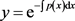$G\left(x,\xi \right)=\left\{\begin{array}{l}{c}_{1}{\text{e}}^{-\int p\left(x\right)\text{d}x},a\xi \end{array}$

$G\left(x,\xi \right)=\left\{\begin{array}{l}0,a

$G\left(x,\xi \right)=\left\{\begin{array}{l}0,a\xi \end{array}$

$G\left(x,\xi \right)={\text{e}}^{-{\int }_{\xi }^{x}p\left(t\right)\text{d}t}H\left(x-\xi \right)$

6.3. 二阶非齐次常微分方程

$\left\{\begin{array}{l}L\left[y\right]={y}^{″}+p\left(x\right){y}^{\prime }+q\left(x\right)y=f\left(x\right),x\in \left(a,b\right)\\ y\left(a\right)=y\left(b\right)=0\end{array}$

$\left\{\begin{array}{l}L\left[G\left(x,\xi \right)\right]=\delta \left(x-\xi \right)\\ G\left(a,\xi \right)=G\left(b,\xi \right)=0\end{array}$ (18)

$\begin{array}{c}L\left[{\int }_{a}^{b}G\left(x,\xi \right)f\left(\xi \right)\text{d}\xi \right]={\int }_{a}^{b}L\left[G\left(x,\xi \right)\right]f\left(\xi \right)\text{d}\xi \\ ={\int }_{a}^{b}\delta \left(x-\xi \right)f\left(\xi \right)\text{d}\xi \\ =f\left(x\right)\end{array}$

$\begin{array}{c}{B}_{i}\left[{\int }_{a}^{\infty }G\left(x,\xi \right)f\left(\xi \right)\text{d}\xi \right]={\int }_{a}^{\infty }{B}_{i}\left[G\left(x,\xi \right)\right]f\left(\xi \right)\text{d}\xi \\ ={\int }_{a}^{b}0\cdot f\left(\xi \right)\text{d}\xi \\ =0\end{array}$

$L\left[G\right]=\delta \left(x-\xi \right),{B}_{1}\left[G\right]={B}_{2}\left[G\right]=0$

$L\left[f\right]=f\left(x\right),{B}_{1}\left[y\right]={B}_{2}\left[y\right]=0$

$\delta \left(x\right)$ 进行积分得到赫维赛德函数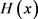$H\left(x\right)={\int }_{-\infty }^{x}\delta \left(t\right)\text{d}t=\left\{\begin{array}{l}0,x<0\\ 1,x>0\end{array}$

$r\left(x\right)={\int }_{-\infty }^{x}H\left(t\right)\text{d}t=\left\{\begin{array}{l}0,x<0\\ x,x>0\end{array}$

$x\ne 0$ 时， $\delta \left(x\right)$ 的导数为0；在 $x=0$ 处，它的导数从0上升到 $+\infty$ ，下降到 $-\infty$ 然后回到0。

${G}^{″}\left(x,\xi \right)+p\left(x\right){G}^{\prime }\left(x,\xi \right)+q\left(x\right)G\left(x,\xi \right)=\delta \left(x-\xi \right)$

${y}_{1},{y}_{2}$ 是齐次微分方程 $L\left[y\right]=0$ 的两个线性无关解。当 $x\ne \xi$ 时，格林函数满足齐次微分方程，因此它是齐次解的线性组合。

$G\left(x,\xi \right)=\left\{\begin{array}{l}{c}_{1}{y}_{1}+{c}_{2}{y}_{2},x<\xi \\ {d}_{1}{y}_{1}+{d}_{2}{y}_{2},x>\xi \end{array}$

$L\left[G\left(x,\xi \right)\right]=\delta \left(x-\xi \right)$$\left({\xi }^{-},{\xi }^{+}\right)$ 上积分，得

${\int }_{{\xi }^{-}}^{{\xi }^{+}}\left[{G}^{″}\left(x,\xi \right)+p\left(x\right){G}^{\prime }\left(x,\xi \right)+q\left(x\right)G\left(x,\xi \right)\right]\text{d}x={\int }_{{\xi }^{-}}^{{\xi }^{+}}\delta \left(x-\xi \right)\text{d}x$

$\begin{array}{l}{\int }_{{\xi }^{-}}^{{\xi }^{+}}p\left(x\right){G}^{\prime }\left(x,\xi \right)\text{d}x=0,{\int }_{{\xi }^{-}}^{{\xi }^{+}}q\left(x\right)G\left(x,\xi \right)\text{d}x=0,\\ {\int }_{{\xi }^{-}}^{{\xi }^{+}}{G}^{″}\left(x,\xi \right)\text{d}x={\int }_{{\xi }^{-}}^{{\xi }^{+}}\delta \left(x-\xi \right)\text{d}x,\\ ⇒{{G}^{\prime }\left(x,\xi \right)|}_{{\xi }^{-}}^{{\xi }^{+}}={\left[H\left(x-\xi \right)\right]|}_{{\xi }^{-}}^{{\xi }^{+}},\\ ⇒{G}^{\prime }\left({\xi }^{+},\xi \right)-{G}^{\prime }\left({\xi }^{-},\xi \right)=1.\end{array}$

${d}_{1}{{y}^{\prime }}_{1}\left(\xi \right)+{d}_{2}{{y}^{\prime }}_{2}\left(\xi \right)-{c}_{1}{{y}^{\prime }}_{1}\left(\xi \right)-{c}_{2}{{y}^{\prime }}_{2}\left(\xi \right)=1$

6.4. 斯特姆-刘维尔问题

$L\left[y\right]=\frac{\text{d}}{\text{d}x}\left[p\left(x\right)\frac{\text{d}y}{\text{d}x}\right]+q\left(x\right)y=f\left(x\right)$ (19)

$L\left[G\left(x,\xi \right)\right]=\delta \left(x-\xi \right),{B}_{1}\left[G\left(x,\xi \right)\right]={B}_{2}\left[G\left(x,\xi \right)\right]=0$

${y}_{1},{y}_{2}$ 是方程(19)对应的齐次方程的两个非零解(分别满足左、右边值条件)，即

$\left\{\begin{array}{l}L\left[{y}_{1}\right]=0\\ {B}_{1}\left[{y}_{1}\right]=0\end{array},\left\{\begin{array}{l}L\left[{y}_{2}\right]=0\\ {B}_{2}\left[{y}_{2}\right]=0\end{array}$

$x\ne \xi$ 时，格林函数满足的微分方程退化为齐次方程，因此可以改写如下形式

$G\left(x,\xi \right)=\left\{\begin{array}{l}{c}_{1}\left(\xi \right){y}_{1}\left(x\right),a\le x\le \xi \\ {c}_{2}\left(\xi \right){y}_{2}\left(x\right),\xi \le x\le b\end{array}$

$\begin{array}{l}G\left({\xi }^{-},\xi \right)=G\left({\xi }^{+},\xi \right)\\ {c}_{1}\left(\xi \right){y}_{1}\left(\xi \right)={c}_{2}\left(\xi \right){y}_{2}\left(\xi \right)\end{array}$

${G}^{″}\left(x,\xi \right)+\frac{{p}^{\prime }}{p}{G}^{\prime }\left(x,\xi \right)+\frac{q}{p}G\left(x,\xi \right)=\frac{\delta \left(x-\xi \right)}{p}$

$\begin{array}{l}{G}^{\prime }\left({\xi }^{+},\xi \right)-{G}^{\prime }\left({\xi }^{-},\xi \right)=\frac{1}{p\left(\xi \right)},\\ {c}_{2}\left(\xi \right){{y}^{\prime }}_{2}\left(\xi \right)-{c}_{1}\left(\xi \right){{y}^{\prime }}_{1}\left(\xi \right)=\frac{1}{p\left(\xi \right)},\end{array}$

$\left\{\begin{array}{l}{c}_{1}\left(\xi \right){y}_{1}\left(\xi \right)-{c}_{2}\left(\xi \right){y}_{2}\left(\xi \right)=0\\ {c}_{1}\left(\xi \right){{y}^{\prime }}_{1}\left(\xi \right)-{c}_{2}\left(\xi \right){{y}^{\prime }}_{2}\left(\xi \right)=-\frac{1}{p\left(\xi \right)}\end{array}$

${c}_{1}\left(\xi \right)=-\frac{{y}_{2}\left(\xi \right)}{p\left(\xi \right)\left(-W\left(\xi \right)\right)},{c}_{2}\left(\xi \right)=-\frac{{y}_{1}\left(\xi \right)}{p\left(\xi \right)\left(-W\left(\xi \right)\right)}$

$G\left(x,\xi \right)=\left\{\begin{array}{l}\frac{{y}_{1}\left(x\right){y}_{2}\left(\xi \right)}{p\left(\xi \right)W\left(\xi \right)},a\le x\le \xi \\ \frac{{y}_{2}\left(x\right){y}_{1}\left(\xi \right)}{p\left(\xi \right)W\left(\xi \right)},\xi \le x\le b\end{array}$

6.5. 初值问题

$\left\{\begin{array}{l}L\left[y\right]={y}^{″}+p\left(x\right){y}^{\prime }+q\left(x\right)y=f\left(x\right),x\in \left(a,b\right)\\ y\left(a\right)={y}_{1},{y}^{\prime }\left(a\right)={y}_{2}\end{array}$

${u}^{″}+p\left(x\right){u}^{\prime }+q\left(x\right)u=f\left(x\right),u\left(a\right)=0,{u}^{\prime }\left(a\right)=0$

${u}^{″}+p\left(x\right){u}^{\prime }+q\left(x\right)u=0,u\left(a\right)={\gamma }_{1},{u}^{\prime }\left(a\right)={\gamma }_{2}$

$W\left(x\right)=c\mathrm{exp}\left(-\int p\left(x\right)\text{d}x\right)$

$\left\{\begin{array}{l}{G}^{″}\left(x,\xi \right)+p\left(x\right){G}^{\prime }\left(x,\xi \right)+q\left(x\right)G\left(x,\xi \right)=\delta \left(x-\zeta \right)\\ G\left(a,\xi \right)=0,{G}^{\prime }\left(a,\xi \right)=0\end{array}$

$G\left({\xi }^{-},\xi \right)=G\left({\xi }^{+},\xi \right),{G}^{\prime }\left({\xi }^{-},\xi \right)+1={G}^{\prime }\left({\xi }^{+},\xi \right)$

${u}_{1},{u}_{2}$ 为微分方程的两个线性无关解，当 $x<\xi$ 时， $G\left(x,\xi \right)$ 是这些解的线性组合，因为伏朗斯基行列式非零，故只有平凡解满足齐次初值条件，格林函数为

$G\left(x,\xi \right)=\left\{\begin{array}{l}0,x<\xi \\ {u}_{\xi }\left(x\right),x>\xi \end{array}$

${u}_{\xi }\left(\xi \right)=0,{{u}^{\prime }}_{\xi }\left(\xi \right)=1$

$G\left(x,\xi \right)=H\left(x-\xi \right){u}_{\xi }\left(x\right)$

$\begin{array}{c}u={\int }_{a}^{b}G\left(x,\text{}\xi \right)f\left(\xi \right)\text{d}\xi \\ ={\int }_{a}^{b}H\left(x-\xi \right){u}_{\xi }\left(x\right)f\left(\xi \right)\text{d}\xi \\ ={\int }_{a}^{x}{u}_{\xi }\left(x\right)f\left(\xi \right)\text{d}\xi \end{array}$

$y=v+{\int }_{a}^{x}{u}_{\xi }\left(x\right)f\left(\xi \right)\text{d}\xi$

6.6. 混合边值问题

$\left\{\begin{array}{l}{B}_{1}\left[y\right]={\alpha }_{11}y\left(a\right)+{\alpha }_{12}{y}^{\prime }\left(a\right)+{\beta }_{11}y\left(b\right)+{\beta }_{12}{y}^{\prime }\left(b\right)={\gamma }_{1}\\ {B}_{2}\left[y\right]={\alpha }_{21}y\left(a\right)+{\alpha }_{22}{y}^{\prime }\left(a\right)+{\beta }_{21}y\left(b\right)+{\beta }_{22}{y}^{\prime }\left(b\right)={\gamma }_{2}\end{array}$

$\begin{array}{l}{u}^{″}+p\left(x\right){u}^{\prime }+q\left(x\right)u=f\left(x\right),{B}_{1}\left[u\right]={B}_{2}\left[u\right]=0,\\ {v}^{″}+p\left(x\right){v}^{\prime }+q\left(x\right)v=0,{B}_{1}\left[v\right]={y}_{1},{B}_{2}\left[v\right]={y}_{2},\end{array}$

${y}_{1}$${y}_{2}$ 是满足齐次边界条件 ${B}_{1}\left[{y}_{1}\right]=0$${B}_{2}\left[{y}_{2}\right]=0$ 的齐次常微分方程的解，又因为完全齐次问题无解，则 ${B}_{1}\left[{y}_{2}\right]$${B}_{2}\left[{y}_{1}\right]$ 非零。解v有如下形式

$v={c}_{1}{y}_{1}+{c}_{2}{y}_{2}$

$v=\frac{{\gamma }_{2}}{{B}_{2}\left[{y}_{1}\right]}{y}_{1}+\frac{{\gamma }_{1}}{{B}_{1}\left[{y}_{2}\right]}{y}_{2}$

${G}^{″}\left(x,\xi \right)+p\left(x\right){G}^{\prime }\left(x,\xi \right)+q\left(x\right)G\left(x,\xi \right)=\delta \left(x-\xi \right),{B}_{1}\left[G\right]={B}_{2}\left[G\right]=0$

$G\left({\xi }^{-},\xi \right)=G\left({\xi }^{+},\xi \right),{G}^{\prime }\left({\xi }^{-},\xi \right)+1={G}^{\prime }\left({\xi }^{+},\xi \right)$

$G\left(x,\xi \right)=H\left(x-\xi \right){y}_{\xi }\left(x\right)+{c}_{1}{y}_{1}\left(x\right)+{c}_{2}{y}_{2}\left(x\right)$

$\begin{array}{l}{B}_{1}\left[G\right]={B}_{1}\left[H\left(x-\xi \right){y}_{\xi }\right]+{c}_{2}{B}_{1}\left[{y}_{2}\right]=0,\\ {B}_{2}\left[G\right]={B}_{2}\left[H\left(x-\xi \right){y}_{\xi }\right]+{c}_{1}{B}_{2}\left[{y}_{1}\right]=0,\end{array}$

$G\left(x,\xi \right)=H\left(x-\xi \right){y}_{\xi }\left(x\right)-\frac{{\beta }_{21}{y}_{\xi }\left(b\right)+{\beta }_{22}{{y}^{\prime }}_{\xi }\left(b\right)}{{B}_{2}\left[{y}_{1}\right]}{y}_{1}\left(x\right)-\frac{{\beta }_{11}{y}_{\xi }\left(b\right)+{\beta }_{12}{{y}^{\prime }}_{\xi }\left(b\right)}{{B}_{1}\left[{y}_{2}\right]}{y}_{2}\left(x\right)$

$u={\int }_{a}^{b}G\left(x,\xi \right)f\left(\xi \right)\text{d}\xi$

$y={\int }_{a}^{b}G\left(x,\xi \right)f\left(\xi \right)\text{d}\xi +\frac{{\gamma }_{2}}{{B}_{2}\left[{y}_{1}\right]}{y}_{1}+\frac{{\gamma }_{1}}{{B}_{1}\left[{y}_{2}\right]}{y}_{2}$

6.7. 高阶非齐次常微分方程

$L\left[y\right]={y}^{\left(n\right)}+{p}_{n-1}\left(x\right){y}^{\left(n-1\right)}+\cdots +{p}_{1}\left(x\right){y}^{\prime }+{p}_{0}y=f\left(x\right)$

${B}_{j}\left[y\right]={y}_{j}$

$B\left[y\right]=\underset{k=0}{\overset{n-1}{\sum }}{\alpha }_{k}{y}^{\left(k\right)}\left(a\right)+\underset{k=0}{\overset{n-1}{\sum }}{\beta }_{k}{y}^{\left(k\right)}\left(b\right)$

$\begin{array}{l}L\left[u\right]=f\left(x\right),{B}_{j}\left[u\right]=0,\\ L\left[v\right]=0,{B}_{j}\left[v\right]={y}_{j}.\end{array}$

$\left(\begin{array}{cccc}{B}_{1}\left[{y}_{1}\right]& {B}_{1}\left[{y}_{2}\right]& \cdots & {B}_{1}\left[{y}_{n}\right]\\ {B}_{2}\left[{y}_{1}\right]& {B}_{2}\left[{y}_{2}\right]& \cdots & {B}_{2}\left[{y}_{n}\right]\\ ⋮& ⋮& \ddots & ⋮\\ {B}_{n}\left[{y}_{1}\right]& {B}_{n}\left[{y}_{2}\right]& \cdots & {B}_{n}\left[{y}_{n}\right]\end{array}\right)\left(\begin{array}{c}{c}_{1}\\ {c}_{2}\\ ⋮\\ {c}_{n}\end{array}\right)=\left(\begin{array}{c}{y}_{1}\\ {y}_{2}\\ ⋮\\ {y}_{n}\end{array}\right)$

$\left\{\begin{array}{l}L\left[G\left(x,\xi \right)\right]=\delta \left(x-\xi \right)\\ {B}_{j}\left[G\right]=0\end{array}$

${y}_{\xi }\left(x\right)$ 是满足下列条件的齐次解的组合：

$\begin{array}{l}{y}_{\xi }\left(\xi \right)=0,\\ {{y}^{\prime }}_{\xi }\left(\xi \right)=0,\\ \text{\hspace{0.17em}}\text{\hspace{0.17em}}\text{\hspace{0.17em}}\text{\hspace{0.17em}}\text{\hspace{0.17em}}\text{\hspace{0.17em}}⋮\\ {y}_{\xi }^{\left(n-2\right)}\left(\xi \right)=0,\\ {y}_{\xi }^{\left(n-1\right)}\left(\xi \right)=1,\end{array}$

${y}_{c}\left(x\right)=H\left(x-\xi \right){y}_{\xi }\left(x\right)$

$G\left(x,\xi \right)=H\left(x-\xi \right){y}_{\xi }\left(x\right)+{d}_{1}{y}_{1}\left(x\right)+\cdots +{d}_{n}{y}_{n}\left(x\right)$

$\left(\begin{array}{cccc}{B}_{1}\left[{y}_{1}\right]& {B}_{1}\left[{y}_{2}\right]& \cdots & {B}_{1}\left[{y}_{n}\right]\\ {B}_{2}\left[{y}_{1}\right]& {B}_{2}\left[{y}_{2}\right]& \cdots & {B}_{2}\left[{y}_{n}\right]\\ ⋮& ⋮& \ddots & ⋮\\ {B}_{n}\left[{y}_{1}\right]& {B}_{n}\left[{y}_{2}\right]& \cdots & {B}_{n}\left[{y}_{n}\right]\end{array}\right)\left(\begin{array}{c}{d}_{1}\\ {d}_{2}\\ ⋮\\ {d}_{n}\end{array}\right)=\left(\begin{array}{c}-{B}_{1}\left[H\left(x-\xi \right){y}_{\xi }\left(x\right)\right]\\ -{B}_{2}\left[H\left(x-\xi \right){y}_{\xi }\left(x\right)\right]\\ ⋮\\ -{B}_{n}\left[H\left(x-\xi \right){y}_{\xi }\left(x\right)\right]\end{array}\right)$

$u={\int }_{a}^{b}G\left(x,\text{}\xi \right)f\left(\xi \right)\text{d}\xi$

7. 结论

$y={\int }_{a}^{b}G\left(x,\xi \right)f\left(\xi \right)\text{d}\xi$

NOTES

*通讯作者。

  Green, G., Wheelhouse, T. and Lindsay, R.B. (2008) An Essay on the Application of Mathematical Analysis to the Theories of Elec-tricity and Magnetism. Journal Für Die Reine Und AngewandteMathematik, 1850, 73-89.  Poincaré, M.H. (1894) Sur les équations de la physique mathématique. Rendiconti del CircoloMatematico di Palermo, 8, 57-155. https://doi.org/10.1007/BF03012493  Morse, P.M.C. and Feshbach, H. (1946) Methods of Theoretical Physics. Technology Press, New York.  Payne, L.E. and Mackie, A.G. (1966) Boundary Value Problems. American Mathematical Monthly, 73, 910. https://doi.org/10.2307/2314220  Neumann, C. (2009) Ueber die Integration der Partiellen Differentialgleichung: . Journal Für Die Reine Und AngewandteMathematik, 1861, 335-366. https://doi.org/10.1515/crll.1861.59.335  Thomson, W. (2013) On the Theory of the Electric Telegraph. Proceedings of the Royal Society of London, 7, 382-399. https://doi.org/10.1017/CBO9780511996016.009  Cagniard, L. (1935) Diffraction d’uneonde Progressive par un Écranenforme de Demi-plan. Journal de Physique et le Radium, 6, 369-372. https://doi.org/10.1051/jphysrad:0193500609036900  Schouten, G. (1999) Two-Dimensional Effects in the Edge Sound of Vortices and Dipoles. The Journal of the Acoustical Society of America, 106, 3167-3177. https://doi.org/10.1121/1.428171  Turner, R.D. (1956) The Diffraction of a Cylindrical Pulse by a Half-Plane. Stichting Mathematisch Centrum Toegepaste Wiskunde, 14, 1-12. https://doi.org/10.1090/qam/78911  Lowan, D.A. (1938) VI. On the Operational Determination of Green’s Functions in the Theory of Heat Conduction. Philosophical Magazine, 24, 62-70. https://doi.org/10.1080/14786443708561890  Carrier, G.F. and Pearson, C.E. (1976) CE Partial Differential Equations. Academic Press, New York.  Burkhardt, H. (1894) Sur les Fonctions de Green Relatives à un Domaine d’une Dimension. Bulletin de la Société Mathématique de France, 22, 71-75. https://doi.org/10.24033/bsmf.484  Bôcher, M. (1901) Green’s Functions in Space of One Dimension. Bulletin of the American Mathematical Society, 7, 297-299. https://doi.org/10.1090/S0002-9904-1901-00802-6  Whyburn, W.M. (1924) An Extension of the Definition of the Green’s Function in One Dimension. Annals of Mathematics, 26, 125-130. https://doi.org/10.2307/1967748  王定江. 应用偏微分方程[M]. 杭州: 浙江大学出版社, 2007: 113-116.  Onida, G., Reining, L. and Rubio, A. (2002) Electronic Excitations: Densi-ty-Functional versus Many-Body Green’s-Function Approaches. Reviews of Modern Physics, 74, 601-659. https://doi.org/10.1103/RevModPhys.74.601  闵琦. δ函数的定义及其性质[J]. 大学物理, 2004(9): 18-20.  Barton, G. (1989) Elements of Green’s Functions and Propagation: Potentials, Diffusion, and Waves. Oxford University Press, Oxford, 3-5.  Duffy, D.G. (1982) Green’s Functions with Applications. Second Edition, CRC Press, New York, 20-21.  Armand, G., Manson, J.R. and Jayanthi, C.S. (1986) Thermal Attenuation in Atom-Surface Scattering: The Multiphonon Contribution. Physical Review B Condensed Matter, 34, 6627.  Roach, G. (1982) Green’s Function. Cambridge University Press, Cambridge, 2-3.  Stakgold, I. (1998) Green’s Functions and Boundary Value Problems. Wiley, New York.  Javadpour, S.H. and Ahmadi, A.R. (1993) An Introduction to Ordinary and Partial Differential Equations. Springer, Cham.  Yang, X. (2003) Green’s Function and Positive Solutions for Higher-Order ODE. Applied Mathematics and Computation, 136, 379-393. https://doi.org/10.1016/S0096-3003(02)00056-5  姜礼尚, 孔德兴, 陈志浩. 应用偏微分方程讲义[M]. 北京: 高等教育出版社, 2011: 38-42.